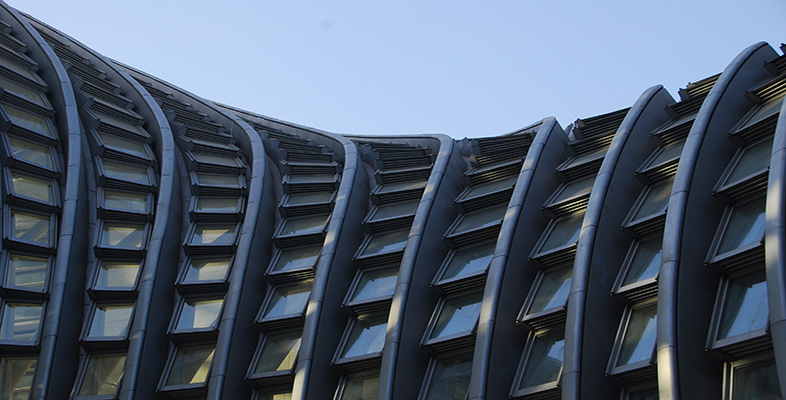Science, Maths & Technology

### Become an OU studentWorking mathematically

Start this free course now. Just create an account and sign in. Enrol and complete the course for a free statement of participation or digital badge if available.

# Appendix: processes uncovered

The following listing provides possible components for the six processes (Source: Begg, 1994, pp. 183–92).

## Problem solving (including investigating)

 (i) planidentify, describe and solve problems; inquire, explore, generate, design, measure and make; formulate a plan and identify sub-tasks; decide whether sufficient information is known; locate, gather and retrieve information; distinguish between important and irrelevant information. (ii) strategiesguess and check; make a list; draw a picture, table or graph; find a pattern, a relationship, and/or a rule; make a model; solve a simpler problem first; work backwards; eliminate possibilities; try extreme cases; write a number sentence; act out a problem; restate the problem; check for hidden assumptions; change the point of view; recognise appropriate procedures and justify them.

## Mathematical modelling

 (i) generaluse concrete materials; use Cartesian and other graphs to model change; use lines, networks, and tree diagrams to represent relationships and sequences; use flow diagrams to represent procedures; use formulae to model relationships; use diagrams and three dimensional models to model geometric situations; andapply the process of mathematical modelling to real world problems. (ii) translatingrestate the real problem as a mathematical problem; use estimation to check the solution; verify and interpret results with respect to the original question; and recognise that the best maths solution may not be the best real solution.

## Reasoning

 (i) classification and description sort and classify objects; describe objects and procedures unambiguously and give definitions; andorganise information to support logic and reasoning.(ii) inferringmake and evaluate mathematical conjectures; infer, interpolate, and extrapolate; make and test hypotheses; make generalisations; and make appropriate and responsible decisions).(iii) reasoningdraw logical conclusions; use models, facts, properties and relationships to provide reasons; justify answers and procedures; use patterns and relationships to analyse situations; develop confidence with spatial reasoning; develop confidence with graphical reasoning (interpretation of graphs); recognise the meanings of true, false and not proven; follow logical arguments; judge the validity of arguments; construct simple valid arguments; recognise and apply deductive reasoning; recognise and apply inductive reasoning; formulate counter examples; appreciate the pervasive use and power of reasoning as a part of mathematics. (iv) proving appreciate the axiomatic nature of mathematics; and construct proofs for mathematical assertions including indirect and inductive proofs.

## Communicating

 (i) personalrelate to others; andwork cooperatively.(ii) general understand what needs to be done in broad terms; reflect on and clarify thinking; relate everyday language to mathematical language, understand mathematical vocabulary; formulate definitions; express generalisations.(iii) listening and speakingfollow instructions from the teacher; discuss difficulties; ask questions; present and explain results to others; discuss the implications and accuracy of conclusions; discuss other possible interpretations of conclusions. (iv) reading and writing follow instructions from a text or a computer; debate possible courses of action with others; use reference material; and make a report.(v) representinguse graphs and diagrams to depict mathematical ideas visually; use symbols to represent ideas precisely; explore problems and describe results using graphical, numerical, physical, algebraic, and verbal mathematical models or representations; appreciate the economy, power, and elegance of mathematical notation.

## Making connections

 (i) within mathematicslink concepts, procedures, and topics in mathematics; relate various representations of a concept or procedure to one another; recognise equivalent representations; see mathematics as an integrated whole.(ii) other curriculum areasuse mathematics in other school subjects (iii) everyday lifeuse mathematics in everyday life, in work and leisure activities; relate results to one’s everyday experienced world.(iv) general apply mathematics to familiar and unfamiliar situations; value the relationship between mathematics and history, culture and society.

## Using tools

 (i) instruments use measuring and drawing instruments.(ii) calculators use simple, scientific, graphical, symbol manipulating calculators. (iii) computers use computers, and general applications such as word processing, databases, spreadsheets; use the Web as a resource for information; use specialist packages for handling and graphing data, for symbol manipulation and graphing, and for dynamic geometry.